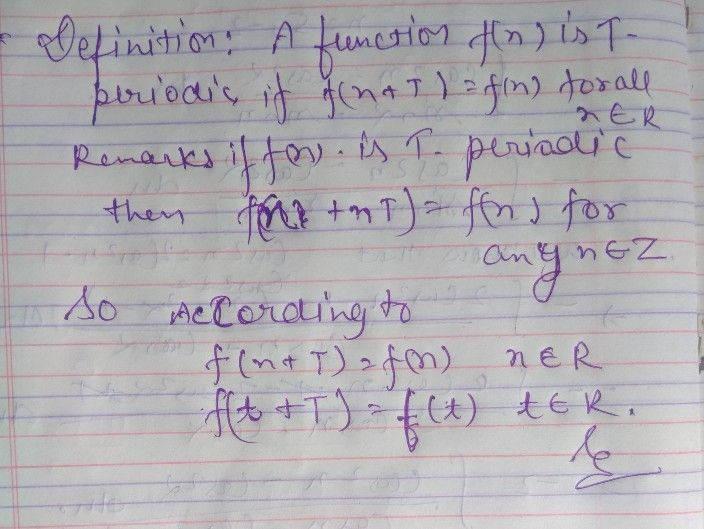Symbol
ProblemQ. In Fourier Series, a periodic $2$ points function is given by a function which $k$ O has a period $2$ pi O satisfies $f\left(t+T\right)=f\left(t\right)$ O satisfies $f\left(t+T\right)=-f\left(t\right)$ O has a period pi
Calculus
Search count: 105
SolutionQanda teacher - ShaliniVeif you have further any doubt ask me please let me know
option B is right
you can also ask Question in preferred by liking my profile